### FLOOR Function – Examples in Excel, VBA, Google Sheets

This tutorial demonstrates how to use the Excel FLOOR Function in Excel to round a number down.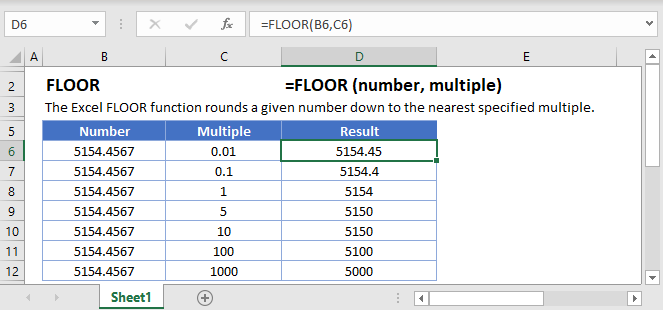## FLOOR Function Description:

The FLOOR Function Rounds a number down, to the nearest specified multiple.

To use the FLOOR Excel Worksheet Function, select a cell and type: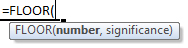(Notice how the formula inputs appear)

## FLOOR Function Syntax and Inputs:

`=FLOOR(number,significance)`

number – A number.

significance – The multiple to which to round the number. The sign much match the number. So if the number is negative, the significance must also be negative.

AutoMacro - VBA Code Generator

## How to use the FLOOR Function

### Round down to Nearest 5, 50, or .5

To round down to the nearest 5, set the multiple argument to 5.

`=FLOOR(B3,5)`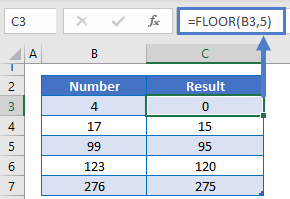Alternatively you can round down to the nearest .5 or 50 by changing the multiple argument.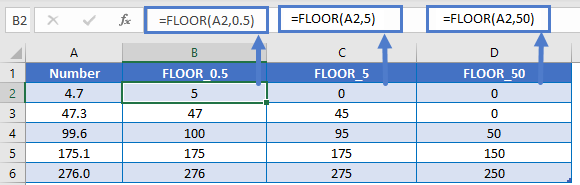### Round down to Nearest Quarter

You can also round a price down to the nearest quarter by setting multiple = .25.

`=FLOOR(A2,0.25)`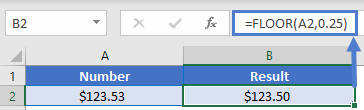### Round Time

The FLOOR Function makes it easy to round down time. To round time down to a certain increment, just enter your desired unit of time using quotations. Here we will round down to the nearest 15 minutes.

`=FLOOR(B3,"0:15")`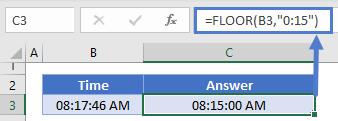## Other ROUND Functions / Formulas

Excel / Google Sheets contains many other round functions. Here are quick examples of each: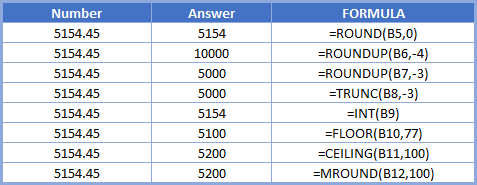Read our tutorials to learn more:

Below, we will point out a few functions in particular.

### CEILING and MROUND

The CEILING and MROUND Functions work exactly the same as the FLOOR Function, except the CEILING Function always rounds up and the MROUND Function always rounds to the nearest multiple.

```=MROUND(B3,5)
=FLOOR(B3,5)
=CEILING(B3,5)```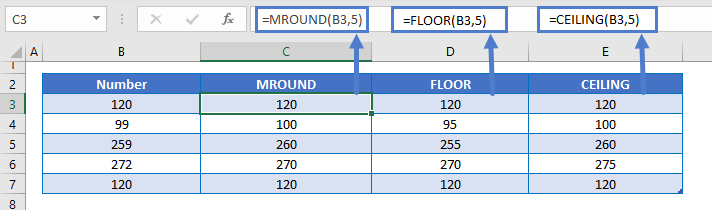### ROUNDDOWN Function

Instead of using the FLOOR Function to round down to a multiple, you can use the ROUNDDOWN Function to round a number down to a certain number of digits.

`=ROUNDDOWN(B3,2)`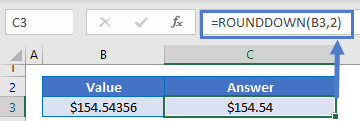## FLOOR in Google Sheets

The FLOOR Function works exactly the same in Google Sheets as in Excel: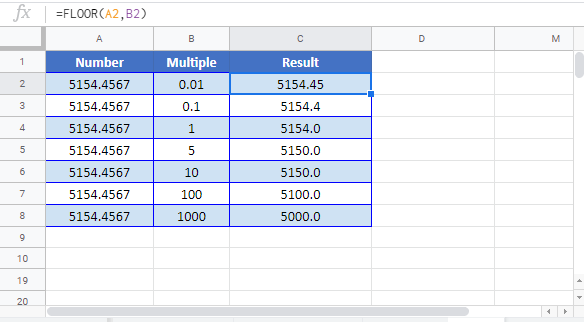## FLOOR Examples in VBA

You can also use the FLOOR function in VBA. Type:` application.worksheetfunction.floor(number,significance)`

Running the following VBA statements

```Range("C2") = Application.WorksheetFunction.Floor(Range("A2"), Range("B2"))
Range("C3") = Application.WorksheetFunction.Floor(Range("A3"), Range("B3"))
Range("C4") = Application.WorksheetFunction.Floor(Range("A4"), Range("B4"))
Range("C5") = Application.WorksheetFunction.Floor(Range("A5"), Range("B5"))
Range("C6") = Application.WorksheetFunction.Floor(Range("A6"), Range("B6"))```

will create the following result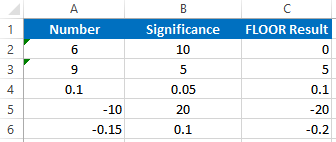For the function arguments (number, etc.), you can either enter them directly into the function, or define variables to use instead.

Return to the List of all Functions in Excel

## Excel Practice Worksheet

Practice Excel functions and formulas with our 100% free practice worksheets!

• Automatically Graded Exercises
• Learn Excel, Inside Excel!